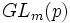# Every group of prime power order is a subgroup of a group of unipotent upper-triangular matrices

This article gives the statement, and possibly proof, of an embeddability theorem: a result that states that any group of a certain kind can be embedded in a group of a more restricted kind.
View a complete list of embeddability theorems

## Statement

Suppose$G$ is a Group of prime power order (?), i.e., a group of order$m = p^n$ for some prime$p$. Then,$G$ is isomorphic to a subgroup of$U_m(p)$, where$U_m(p)$ is the group of upper-triangular$m \times m$ matrices with$1$s on the diagonal, over the prime field$F_p$.

## Facts used

• Cayley's theorem
• Sylow implies order-dominating: The domination part of Sylow's theorem, which states that given a$p$-subgroup and a$p$-Sylow subgroup, some conjugate of the$p$-subgroup lies inside the$p$-Sylow subgroup.

## Proof

Given: A group$G$ of order$m = p^n$ for some natural number$n$.

To prove:$G$ can be embedded as a subgroup of$U_m(p)$, the group of upper triangular unipotent$m \times m$ matrices over the field of$p$ elements.

Proof: By Cayley's theorem (fact (1)),$G$ is a subgroup of the symmetric group on$m$ elements. This, in turn, is a subgroup of the general linear group$GL_m(p)$, under the embedding that sends each permutation to its corresponding permutation matrix. Thus,$G$ embeds as a$p$-subgroup of$GL_m(p)$.

Now, the group$U_m(p)$ is a$p$-Sylow subgroup of$GL_m(p)$, so by fact (2), some conjugate of$G$ lies inside$U_m(p)$. Since this conjugate subgroup is in particular isomorphic to$G$, we obtain an embedding of$G$ as a subgroup of$U_m(p)$.